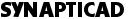# v2vh_tap.v

```/////////////////////////////////////////////////////
// tap_controller component description
/////////////////////////////////////////////////////

`timescale 1ns/1ns

module tap (\out ,_enable_,register,re\$etn,\***shift***ir*** ,with,
clock_ir,\shift+dr ,clock_,capture__dr,abs,
now,tck,in);

output \out ,_enable_,register,re\$etn,\***shift***ir*** ,with,
clock_ir,\shift+dr ,clock_,capture__dr,abs;
input now,tck,in;

reg re\$etn,_enable_,\***shift***ir*** ,\shift+dr ;
reg D,C,B,A;

wire inhi,tckd,NA,NB,NC,ND,GRST,GSIR,GSDR,GENB;

initial
begin
D = 1;
C = 1;
B = 1;
A = 1;
end

buf ibuf (buffer,now);
not ntobuf1 (inhi, in), notbuf2(tckd, tck);
and #2 TLR(register, A, B, C, D);

//concurrent statements to define output logic

assign (strong1, strong0) \out  = D;
assign clock_ir = ~(~A & B & D & tckd);
assign with = A & ~B & C & D & tckd;
assign clock_ = ~(~A & B & ~D & tckd);
assign abs = A & ~B & C & ~D & tckd;
assign capture__dr = ~A & B & C & ~D;

//concurrent statements to define next state logic

assign NA = ~((~(~buffer & (~C & A)) & (~(buffer & ~B))) &
(~(buffer & ~A) & ~(buffer & (C & D))) );

assign NB = ~(((((( ~(~buffer & (B & ~A)) & ~(~buffer & ~C)) &
~(~buffer & (~D & B))) &
~(~buffer & (~D & ~A))) &
~(buffer & (C & ~B))) &
~((buffer & D) & (C & A))) );

assign NC = ~(~(C & ~B) & ~(C & A) & ~(buffer & ~B) );

assign ND = ~((~(D & ~C) & ~(D & B)) &
(~(~buffer & (C & ~B)) &
~((~D & C) & (~B & ~A))) );

//combinational logic

assign GRST = ~((A & B) & (C & D));
assign GSIR = (~A & B) & (~C & D);
assign GSDR = (~A & B) & (~C & ~D);
or or1(GENB, GSIR, GSDR);

always @(posedge tckd or posedge inhi)
begin
if (inhi==1)
re\$etn = 0;
else
re\$etn = GRST;
end

always @(posedge tckd or posedge inhi)
begin
if (inhi==1)
_enable_ = 1'b0;
else
_enable_ = GENB;
end

always @(posedge tckd or posedge inhi)
begin
if (inhi==1)
\***shift***ir*** = 1'b1;
else
\***shift***ir*** = GSIR;
end

always @(posedge tckd or posedge inhi)
begin
if (inhi==1'b1)
\shift+dr = 1'b1;
else
\shift+dr = GSDR;
end

always @(posedge tck or posedge inhi)
begin
if (inhi==1)
D = 1;
else if (tck==1)
D <= #1 ND;
end

always @(posedge tck or posedge inhi)
begin
if (inhi==1)
C = 1;
else if (tck==1)
C <= #1 NC;
end

always @(posedge tck or posedge inhi)
begin
if (inhi==1)
B = 1;
else if (tck==1)
B <= #1 NB;
end

always @(posedge tck or posedge inhi)
begin
if (inhi==1)
A = 1'b1;
else if (tck==1)
A <= #1 NA;
end

endmodule
```